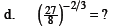### Home > PC > Chapter 1 > Lesson 1.3.1 > Problem1-102

1-102.
1.Add the rules from the Math Notes box to your Tool Kit. It does not matter how you “break up” the fractional exponent. To evaluate fractional exponents without a calculator, make the numbers small as soon as you can by taking the root first and then raising to the power. Homework Help ✎

Evaluate.

1. 642/3 = (641/3)2 = ?

2. 1254/3 = (1251/3)4 = ?

3. 813/4 = ?

4.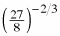= ?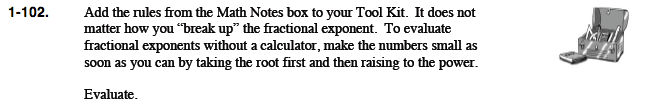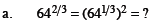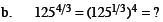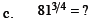$\frac{4}{9}$